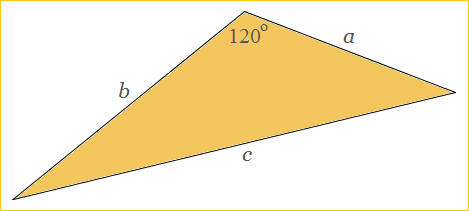# Integer Triangles with a 120° Angle

Just as there are integer right triangles, so there are integer 120° triangles. In such triangles, all side lengths are whole numbers and the obtuse angle measures exactly 120°.

If the two sides adjacent to the 120° angle are a and b and the third side is c, then a, b, and c satisfy a Pythagorean-like relation:

a² + ab + b² = c²,

which holds for all 120° triangles, not just the integer ones.To find triples (a, b, c) that satisfy the relation a² + ab + b² = c², you can use generator functions similar to the generator functions for Pythagorean triples. In the 120° case, the equations are

a = m² - n²
b = 2mn + n²
c = m² + mn + n²,

where m and n are integers and m > n. Observe:

(m² - n²)² + (m² - n²)(2mn + n²) + (2mn + n²)² = (m² + mn + n²)².

In this way you can come up with infinitely many triples (a, b, c). The first few primitive triples (those in which a, b, and c have no factors in common) are given in the table below:

 a b c 3 5 7 7 8 13 5 16 19 11 24 31 7 33 37 13 35 43 16 39 49 9 56 61 32 45 67 17 63 73 40 51 79 11 85 91 19 80 91 55 57 97

The first few triples with consecutive integer side lengths are

(7, 8, 13)
(104, 105, 181)
(1455, 1456, 2521)
(20272, 20273, 35113).